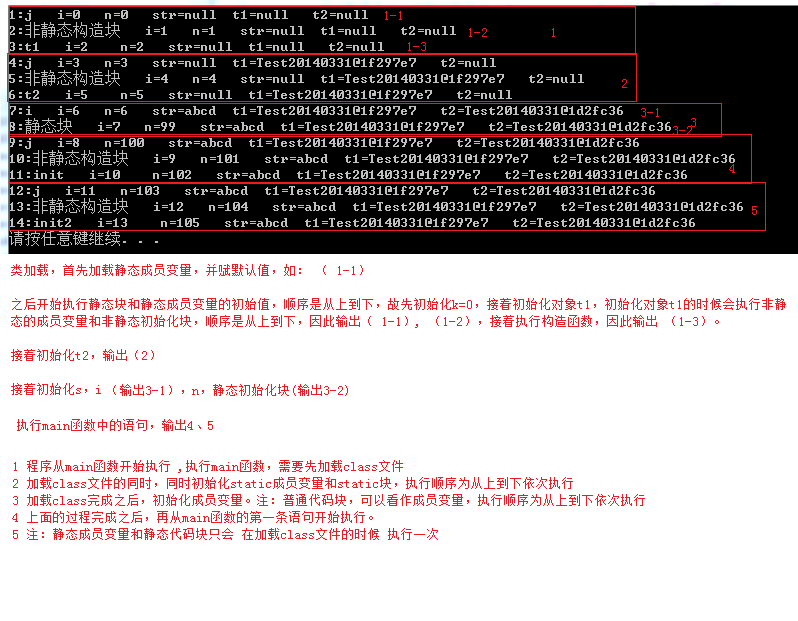public class ExA {

``````private static ExA a = new ExA();
static {
System.out.println("父类--静态代码块");
}

public ExA() {
System.out.println("父类--构造函数");
}

{
System.out.println("父类--非静态代码块");
}

public static void main(String[] args) {
new ExB();
}
``````

}

class ExB extends ExA {

``````private static ExB b = new ExB();
static {
System.out.println("子类--静态代码块");
}
{
System.out.println("子类--非静态代码块");
}

public ExB() {
System.out.println("子类--构造函数");
}
``````

}

5个回答

`````` 父类--非静态代码块

``````

`````` public class ExA {

static {
System.out.println("父类--静态代码块");
}

public static void main(String[] args) {

}

}
``````zyf4005 那么我的疑问是你既然说“我们在使用一个类之前，它必须经过装载，连接，初始化这样的过程。然后我们才能使用new操作实例化创建对象，”那么代码的第一句private static ExA a = new ExA();这时候类ExA还没有加载和初始化完成呢，那岂不是就无法new ExA()了吗？

（且只执行一次，执行的是相关的静态代码块或函数）zyf4005 本来执行private static ExA a = new ExA();的时候因为是首次new,所以会导致类ExA的加载和初始化。因为此时有main导致的类的加载和初始化。这两个初始化是一个类，所以才去执行了非静态代码。那你的意思可以理解为在类的加载和初始化过程中就可以创建对象，没有要求说：类必须加载和初始化完成后才能创建对象是吧？

``````public class Test20160509 {
public static int k = 5;
public static Test20160509 t1 = new Test20160509("t1");
public static Test20160509 t2 = new Test20160509("t2");
public static String s = "abcd";
public static int i = print("i");
public static int n = 99;

public int j = print("j");

{
print("非静态构造块");
}

static {
print("静态块");
}

public Test20160509(String str) {
System.out.println((++k) + ":" + str + "   i=" + i + "    n=" + n + "   str=" + s + "  t1=" + t1 + "   t2=" + t2);
++i;
++n;
}

private static int print(String str) {
System.out.println((++k) + ":" + str + "   i=" + i + "   n=" + n + "   str=" + s + "  t1=" + t1 + "   t2=" + t2);
++n;
return ++i;
}

public static void main(String[] args) {
Test20160509 t = new Test20160509("init");
Test20160509 t2 = new Test20160509("init2");

}

}

``````